# Gamma-function

(diff) ← Older revision | Latest revision (diff) | Newer revision → (diff)-function

A transcendental function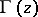that extends the values of the factorialto any complex number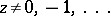. It was introduced in 1729 by L. Euler in a letter to Ch. Goldbach, using the infinite product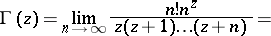which was used by L. Euler to obtain the integral representation (Euler integral of the second kind, cf. Euler integrals)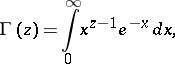which is valid for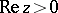. The multi-valuedness of the function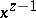is eliminated by the formula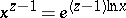with a real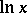. The symboland the name gamma-function were proposed in 1814 by A.M. Legendre.

If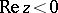and,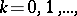the gamma-function may be represented by the Cauchy–Saalschütz integral: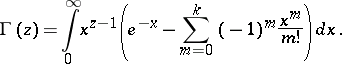In the entire plane punctured at the points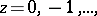the gamma-function satisfies a Hankel integral representation: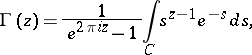whereandis the branch of the logarithm for which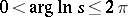; the contouris represented in Fig. a. It is seen from the Hankel representation thatis a meromorphic function. At the points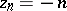,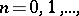it has simple poles with residues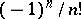.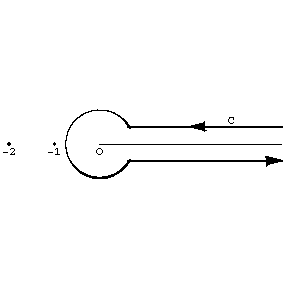Figure: g043310a

## Fundamental relations and properties of the gamma-function.

1) Euler's functional equation: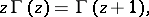or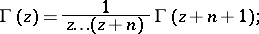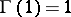,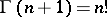if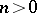is an integer; it is assumed that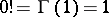.

2) Euler's completion formula: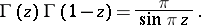In particular,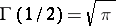;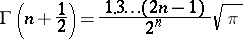ifis an integer;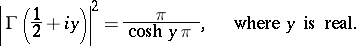3) Gauss' multiplication formula: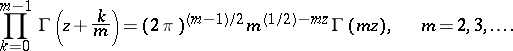If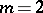, this is the Legendre duplication formula.

4) Ifor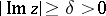, thencan be asymptotically expanded into the Stirling series: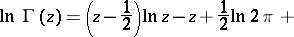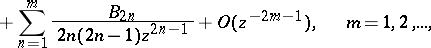where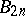are the Bernoulli numbers. It implies the equality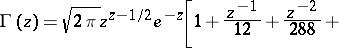In particular,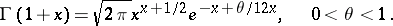More accurate is Sonin's formula :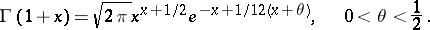5) In the real domain,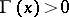for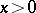and it assumes the signon the segments,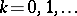(Fig. b).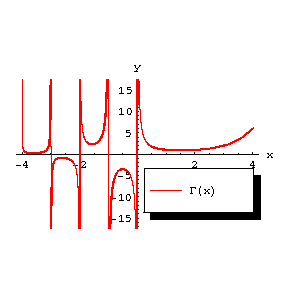Figure: g043310b

The graph of the function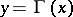.

For all realthe inequality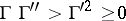is valid, i.e. all branches of both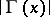andare convex functions. The property of logarithmic convexity defines the gamma-function among all solutions of the functional equation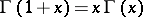up to a constant factor.

For positive values ofthe gamma-function has a unique minimum atequal to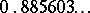. The local minima of the functionform a sequence tending to zero as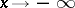.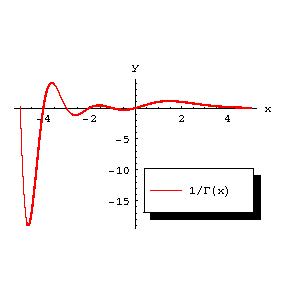Figure: g043310c

The graph of the function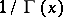.

6) In the complex domain, if, the gamma-function rapidly decreases as,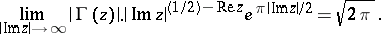7) The function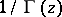(Fig. c) is an entire function of order one and of maximal type; asymptotically, as,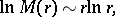where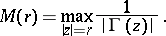It can be represented by the infinite Weierstrass product: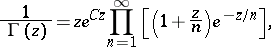which converges absolutely and uniformly on any compact set in the complex plane (is the Euler constant). A Hankel integral representation is valid: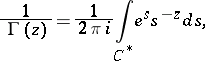where the contour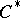is shown in Fig. d.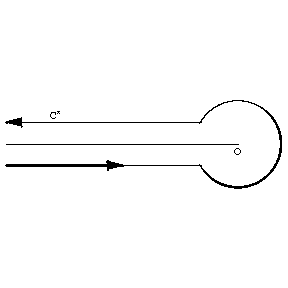Figure: g043310dG.F. Voronoi  obtained integral representations for powers of the gamma-function.

In applications, the so-called poly gamma-functions —-th derivatives of— are of importance. The function (Gauss'-function)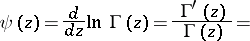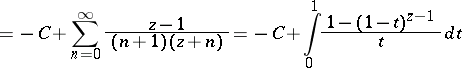is meromorphic, has simple poles at the pointsand satisfies the functional equationThe representation offoryields the formula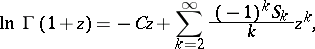where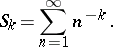This formula may be used to computein a neighbourhood of the point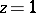.

For other poly gamma-functions see . The incomplete gamma-function is defined by the equation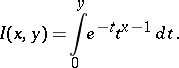The functionsandare transcendental functions which do not satisfy any linear differential equation with rational coefficients (Hölder's theorem).

The exceptional importance of the gamma-function in mathematical analysis is due to the fact that it can be used to express a large number of definite integrals, infinite products and sums of series (see, for example, Beta-function). In addition, it is widely used in the theory of special functions (the hypergeometric function, of which the gamma-function is a limit case, cylinder functions, etc.), in analytic number theory, etc.